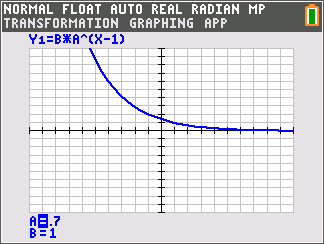••• ##### Device
• TI-84 Plus
• TI-84 Plus Silver Edition
•TI-84 Plus C Silver Edition
•TI-84 Plus CE
• ##### Software

TI Connect™ CE

• ##### Report an Issue

Precalculus: Exploring Geometric Sequences
by Texas Instruments#### Overview

Students graphically analyze geometric series using graphs and consider the effect of the value for the common ratio and first term using sliders.

#### Key Steps

•For the first part of this activity, students explore geometric sequences graphically by varying the value of A, the common ratio. Pressing the right or left arrows will change the value of A and updates the graph each time.

•Changing the values of both of the variables, the first term and common ratio, of a geometric sequence gives students a deeper understanding of their behavior.

•As an extension, student will examine the derivation of the sum of a finite geometric series and use it to solve several problems.